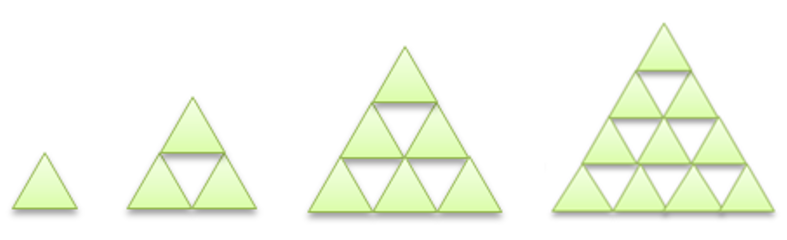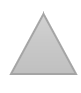Mathematics
Easy

Question

# Sophia made a pattern using triangles.If this pattern continues, the number oftriangles there in the next shape are____.

## 10    14    15    16Hint:

## The correct answer is: 15

### The number of triangles in first shape is 1, second shape is 3, third shape is 9 and fourth shape is 10.First shape has 1 triangle.Second shape has 3 triangle. It has two triangles more that first triangle.Third shape has 6 triangle. It has three more triangles than second.Fourth shape has 10 triangles. It has four more triangles than third.We are asked to find number of triangles in next shape.Fifth shape will have five more triangles.The number of triangles = 10 + 5= 15The number of triangles are 15.

We have to be careful about the order of the pattern.

### Related Questions to study#### With Turito Foundation.#### Get an Expert Advice From Turito.